Clutch Prep is now a part of Pearson
Ch.12 - SolutionsWorksheetSee all chapters

# The Freezing Point Depression (IGNORE)

See all sections
Sections
Solutions: Solubility and Intermolecular Forces
Molality
Parts per Million (ppm)
Mole Fraction
Solutions: Mass Percent
Types of Aqueous Solutions
Intro to Henry's Law
Henry's Law Calculations
The Colligative Properties
Boiling Point Elevation
Freezing Point Depression
Osmosis
Osmotic Pressure
Vapor Pressure Lowering (Raoult's Law)
The Freezing Point Depression (IGNORE)Jules Bruno

The freezing point depression of a solution represents the decrease in the freezing point as a solute is dissolved within a solvent. This results in a lower freezing point for a solution mixture than the pure solvent.

Freezing Point Depression

Phase Diagram

phase diagram can be used to illustrate how the factors of pressure and temperature can influence the three phases of matter for a pure substance.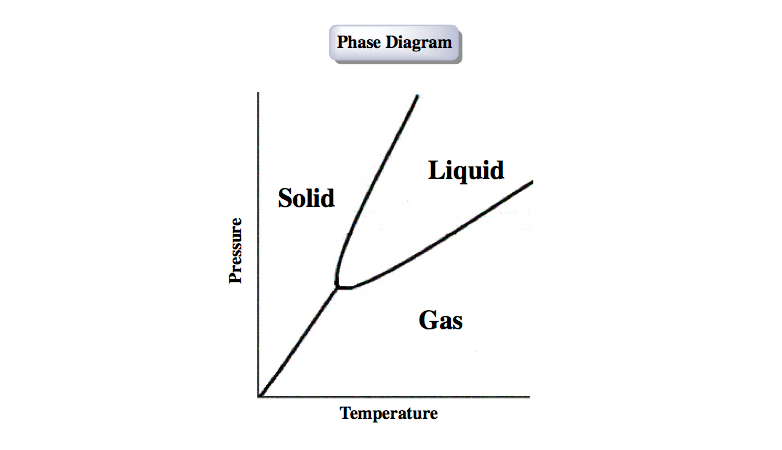Phase Diagram (Solid, liquid, gas)

Within a phase diagram we have a pure substance segmented into the phases of solid, liquid or gas. The transitions between the different phases can be seen on the right of the phase diagram.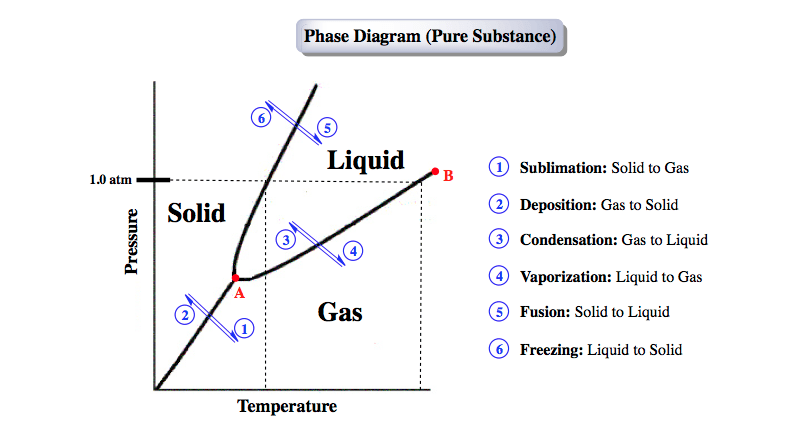Phase Diagram & Phase Transitions

Point A of the phase diagram represents the triple point, the place where all three phases of matter co-exist, and point B represents the critical point, the place where both the liquid and gas phases have the same density and become difficult to tell apart.

The 4 Colligative properties (Boiling Point, Freezing Point, Osmotic Pressure and Vapor Pressure) discuss what happens to a pure solvent as a solute is added to it. This addition of solute to a solvent creates a solution and impacts the phase diagram.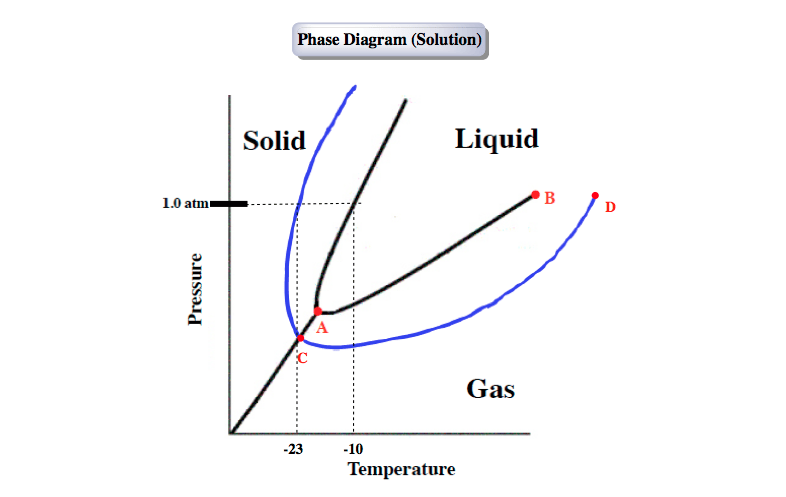Phase Diagram (Solution)

The blue line represents the newly created solution and the original black lines still represent the pure solvent. Following the standard pressure (1.0 atm) and stopping on the line segments for the pure solvent and the solution that represent equilibrium between freezing and melting we can trace downward to find their freezing points. As expected we see that the solution has a lower freezing point (-23°C) than the pure solvent (-10°C).

Van’t Hoff Factor

The Van’t Hoff factor, i, deals with the number of ions your solute dissolves into once it’s dropped into the solvent.

covalent solute is composed of only non-metals and when thrown into an aqueous solvent like water will not break up into ions. This means the covalent solute stays intact and i = 1.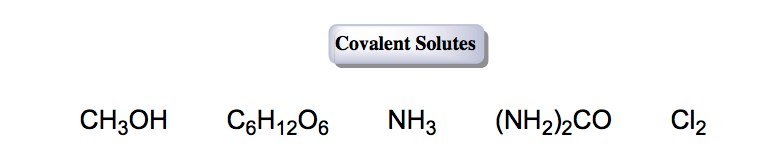Covalent or Non-volatile Solutes

An ionic solute is composed of a cation (positive ion) and an anion (negative ion) and when thrown into an aqueous solvent like water will break up into multiple ions.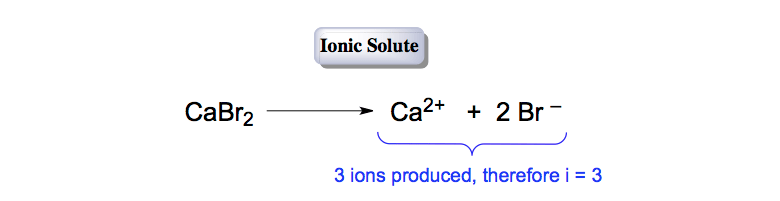Ionic or Volatile Solutes

Freezing Point

Adding solute to a pure solvent causes a decrease in the freezing point. As a result, the freezing point of the solution will be lower than the freezing point of the pure solvent.

To calculate the change in the freezing point (ΔT­f) we use the following equation:Freezing Point Depression Formula

As we mentioned earlier, the variable i represents the Van’t Hoff factor, which is the number of ions your solute dissolves into when placed into a solvent. kf represents the freezing point constant of the pure solvent and m represents the molality of the solution.

Once we calculate the change in the freezing point (ΔT­) then we can determine the new freezing point of the solution.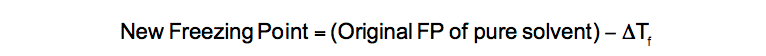Calculating New Freezing Point (Colligative Property)

Now let’s take a look at how to determine the new freezing point of a solution once we add potassium chloride, KCl.

PRACTICE: Calculate the freezing point of a solution containing 25.0 grams of KCl and 2750 grams of water. The Kb and Kf of water are 0.51 °C/m and 1.86 °C/m respectively.

STEP 1: Convert the 25 grams of KCl (solute) into moles.

a) Look up the atomic masses of potassium (K) and chlorine (Cl) from the periodic table to calculate the molar mass of KCl.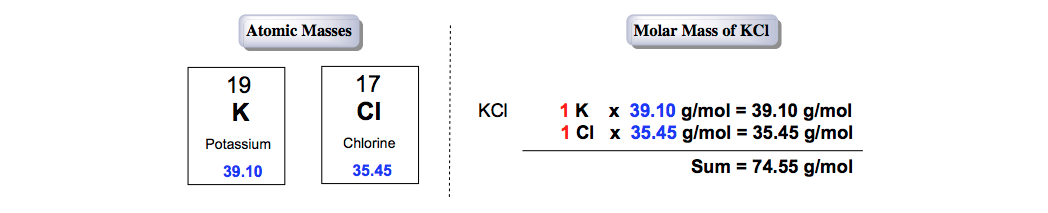Calculating molar mass of KCl

b) Use the molar mass of KCl to find the moles of KCl.Mass conversion of 25.0 g KCl to moles KCl

STEP 2: Convert the 2750 grams of water (solvent) into kilograms.Conversion from 2750 g KCl to kg KCl

STEP 3: Calculate the molality, m, of the solution.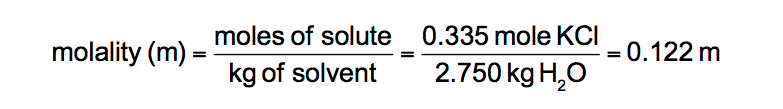Determination of molality (KCl solution)

STEP 4: Determine the van’t Hoff Factor of the solute.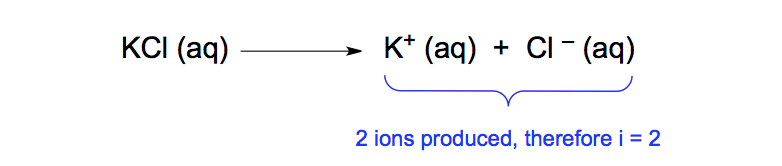Dissociation of KCl solute

STEP 5: Plug the given variables into the Freezing Point Depression Equation to determine the change in the freezing point.Change in Freezing Point  (ΔT­f

STEP 6: Calculate the new freezing point of the solution. Pure water freezes at 0°C.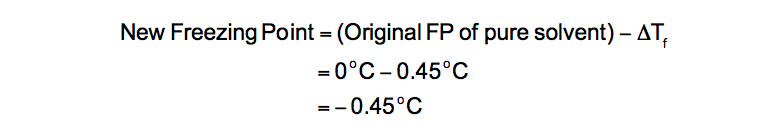Freezing Point of KCl solution

The Other Colligative Properties

Freezing Point Depression represents one of the four colligative properties. The other three colligative properties include Boiling Point Elevation, Osmotic Pressure and Vapor Pressure. All of them are affected when a solute is added to a pure solvent and help to explain the chemical composition and properties of these newly created solutions.Jules Bruno

Jules felt a void in his life after his English degree from Duke, so he started tutoring in 2007 and got a B.S. in Chemistry from FIU. He’s exceptionally skilled at making concepts dead simple and helping students in covalent bonds of knowledge.